eCircuit  Center

### DC Motor Model

CIRCUIT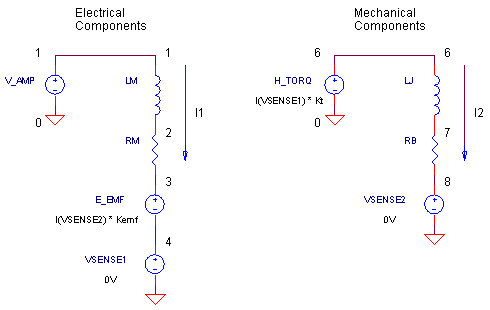Although SPICE does not provide explicit models for electro-mechanical devices, like a DC motor, creating one is fairly straightforward. You just need to remember that most physical behaviors, whether mechanical or electrical, can be described by a set of equations. So for a desired mechanical behavior (inertia, friction, etc.), simply find an equivalent electrical circuit (inductance, resistance, etc.) described by similar equations. Then assign the mechanical parameters to the appropriate electrical component values and you're ready to simulate.

ELECTRICAL WORLD

On the electrical side of the DC motor, a current flows I1 through the armature according to the amplifier's drive voltage VAMP, the motor's inductance LM, resistance RM and the back emf voltage Vemf.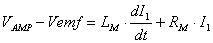In SPICE land, these components are represented by VAMP, RM, LM and E_EMF.

MECHANICAL WORLD

The motor converts the electrical armature current into a mechanical torque applied to the shaft.

T = KT I1

The applied torque produces an angular velocity ω according to the inertia J and friction B of the motor and load.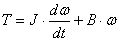However, because inertia and friction don't exist in SPICE, we call in an electrical equivalent - a series RL circuit - described by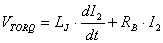Comparing the two equations above, you can see the mechanical and electrical equivalents.

 Parameter Mechanical Electrical » Applied Torque T VTORQ » Velocity w I2 » Inertia J LJ » Friction B RB

In the SPICE circuit, H_TORQ generates a voltage (really torque T ) proportional to the motor current sensed by VSENSE1. Components LJ and RB act as the motors mechanical inertia and friction

BACK EMF

What is the back emf? It's the voltage generated across the motor's terminals as the windings move through the motor's magnetic field. The back emf actually opposes the drive voltage and is proportional to the motor's velocity

Vemf  = Kemf ω

How is this accomplished in SPICE? Voltage source H_EMF generates the back emf based on the current sensed by VSENSE2 (really velocity ω) and multiplies it by the speed constant.

VELOCITY AND POSITION

The last stage of the model simply transforms angular velocity ω, into angular position θ, through the relationComponent FPOS makes a copy of the current in VSENSE2 (really velocity ω) and feeds it to CPOS where it's integrated to get a voltage representing the motor shaft's angular position θ.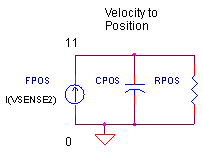DC MOTOR

Okay, let's create a motor model. Here's the parameters at a glance.

 Parameter Definition SPICE Component RA = 0.5 Ω Terminal Resistance RA LA = 1.5 mH Terminal Inductance LA KT = 0.05 Nm / A Torque Constant H_TORQ JM = 0.00025 Nm/rad/s2 Motor and load Inertia JM BM = 0.0001 Nm/rad/s Friction BM Kemf = 0.05 V/rad/s Speed Constant H_EMF

Before we start up the motor, here's a recap of the circuit variables:

V(1)               - Motor Voltage
I(VSENSE1)   - Motor Current
V(3)               - Back emf voltage
V(6)               - Motor Torque
I(VSENSE2)   - Angular Velocity
V(11)             - Angular Position

CIRCUIT INSIGHT   Run the SPICE file DC_MOTOR_MODEL.CIR. VAMP applies a 10 V step voltage across the motor at V(1). Check out the initial inrush of motor current by plotting I(SENSE1). To see how the motor shaft spins, open a new plot window and plot the angular velocity by adding trace I(SENSE2). Although the velocity is plotted in units of A, we know it's really in rad / s. ( For reference: 100 rad /s = 16 rev / s = 955 RPM.) You can watch the back emf voltage grow as velocity increases by plotting V(3) in the original plot window. How much torque is required to achieve this speed? Plot V(6) to see the Nm required.

Look what happens when the 10V voltage is driven down to 0V. The motor slows down. Also notice the motor current, I(VSENSE1), reverses helping to slow down the motor. But why should the motor current reverse, there's no voltage applied. Yes, that's true! But, with 0V applied to the motor, all of the back emf now appears across RM and LM causing the current (and torque) to reverse! This reverse-torque action is referred to as dynamic braking.

What about the angular position of the shaft? Open a new plot window and plot V(11) to see the shaft's position in rad. What do you expect it to look like? As the 10V is initially applied, the position should change slowly at first, then change increasingly faster until it hits full speed where the change in position is constant versus time. When the 10V is removed, the change in position slows down until its stopped.

HANDS-ON DESIGN   Imagine you've bolted a pulley or wheel to the motor shaft resulting in an increased inertia. You can simulate this by increasing the inertia ( LJ ). How much longer does it take to reach full speed?

Want to reach full speed quicker? Buy a motor with a higher torque constant. Increase the constant in the H_TORQ statement from 0.05 to a higher value. You should see a couple of nice effects: First, the motor gets to full speed in less time and second, the duration of the inrush current decreases!

SIMULATION NOTES

A Current-Controlled Voltage Source (CCVS) generates the voltage representing torque.

H_TORQ 6 0 VSENSE1 0.05

according to the equation

VH_TORQ = I(VSENSE1) 0.05

where I(SENSE1) is the current through VSENSE1. You can sense current using any independent voltage source. Typically set to 0V, this source has no effect on the circuit.

A Current-Controlled Current Source (CCCS) makes a copy of the a current representing angular velocity to be integrated into an angular position value.

F_POS 0 11 VSENSE2 1

according to the equation

IFPOS = I(VSENSE2) 1

where I(SENSE2) is the current through independent source VSENSE2..

SPICE FILE

Download the file or copy this netlist into a text file with the *.cir extension.

```DC_MOTOR_MODEL.CIR
*
V_AMP   1 0     AC 1  PWL(0MS 0V  1MS 10V  1000MS 10V  1010MS 0V  2000MS 0V)
*
* MOTOR VOLTAGE
RA	1	2	0.5
LA	2	3	0.0015
H_EMF	3 4	VSENSE2	0.05
VSENSE1	4	0	DC 0V
*
* MOTOR TORQUE BASED ON INERTIA AND FRICTION
H_TORQ	6 0	VSENSE1	0.05
LJ	6	7	0.00025
RB	7	8	0.0001
VSENSE2	8	0	DC	0V
*
* MOTOR POSITION
FPOS	0	11	VSENSE2	1
CPOS	11	0	1
RPOS	11	0	1MEG
*
* ANALYSIS
.TRAN	10MS	2000MS
*
* VIEW RESULTS
.PRINT TRAN V(1) I(VSENSE1)
.PROBE
.END```

Top ↑